•http://facebook.com/
•https://www.google.com/accounts/o8/id
•https://me.yahoo.com

# Internal Combustion Engine Cycles

A discussion on the thermodynamic cycles of internal combustion engines, including the Otto cycle, the Joule cycle, and the Diesel cycle.

## Overview

Definitions

Working substance (WS). The WS is used as the carrier for heat energy. The heat engine carries out the conversion process by a series of changes of state of the WS. The state of the WS is defined by the values of its properties, e.g. pressure, volume, temperature, internal energy, enthalpy. These properties are also sometimes called functions of state.

<br/>

Key facts

Examples of internal combustion engine cycles are the Otto cycle (also known as the Constant Volume cycle), the Joule cycle (also known as the Brayton cycle, or the Constant Pressure cycle), and the Diesel cycle.

The thermal efficiency of the Otto cycle is given by:

where is the compression ratio, and the heat capacity ratio.

The thermal efficiency of the Joule cycle is given by:

where is the pressure ratio, and the heat capacity ratio.

The thermal efficiency of the Diesel cycle is given by:

where is the compression ratio, the expansion ratio during heating (also known as the cut-off ratio), and the heat capacity ratio.

A thermodynamic cycle comprises a series of operations carried out on the working substance (WS) during which heat is supplied, and after which the WS is returned to its original state (for a more comprehensive introduction to thermodynamic cycles also see Thermodynamic Cycles ).

An example of a thermodynamic cycle is the internal combustion engine. In this case, the WS is treated as pure air, the expansions and compressions are reversible and adiabatic, and heat can be added instantaneously if desired. Applications of such cycles are the Otto cycle, the Joule cycle, and the Diesel cycle.

Before discussing these cycles, we first have to introduce the thermal efficiency of a cycle.

The thermal efficiency of a cycle, also denoted by , is a measure of the ability to convert heat energy into work. Therefore, the thermal efficiency can be defined as:

where is the work output and the heat energy supplied. As the work done can also be expressed as:

where is the heat rejected in the WS and in losses (for a more detailed discussion see Thermodynamic Cycles ). The thermal efficiency from (1) becomes:

or furthermore,

## Otto Cycle

The Otto cycle (also sometimes called the Constant Volume cycle) is diagramed on a pressure - volume () plot in Figure 1, and on a temperature - entropy () plot in Figure 2.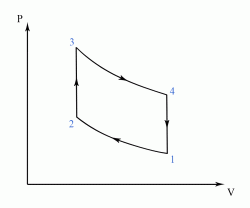Figure 1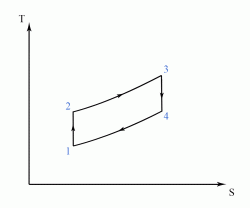Figure 2 This cycle consists of an adiabatic compression of the WS (step ), followed by an isochoric heating, i.e. heating at constant volume (step ), then an adiabatic expansion of the WS (step ), and ended with an isochoric cooling which reverts the system back to its original condition (step ).

We know that the heat added or removed from the WS during a process at constant volume can be written as:

where is the number of moles of the WS, the heat capacity at constant volume, and the change in temperature. Hence, for the Otto cycle depicted in Figure 1 and Figure 2, we have that the heat supplied is:

and the heat rejected is:

Therefore, the thermal efficiency of the Otto cycle becomes (see equation 4):

from which we obtain:

As in this case the WS is treated as pure air, we can write for the adiabatic compression that:

and for the adiabatic expansion that:

In (10) and (11), is the heat capacity ratio defined as:

where is the heat capacity at constant pressure, and the heat capacity at constant volume.

Equations (10) and (11) can also be written as:

and,

respectively. As and (steps and of the Otto cycle are isochoric - see Figure 1), we can rewrite equations (13) and (14) as:

and,

respectively, where is called the compression ratio.

By subtracting equation (15) from equation (16), we get that:

or furthermore, that:

By using (19) in equation (9), we obtain the thermal efficiency of the Otto cycle as:

It can be noted that the compression ratio for carburettor engines is about , while for fuel injector engines it increases to around . By using these figures, and also considering a value for the heat capacity ratio of , the thermal efficiency of a petrol/gasoline engine is found to be between and . It can also be noted that for an Otto cycle engine, the ignition is usually performed by using a spark plug ("spark ignition").

## Joule Cycle

The Joule cycle (also sometimes called the Brayton cycle, or the Constant Pressure cycle) is a thermodynamic cycle that describes the workings of the gas turbine engine. The Joule cycle is depicted on a plot in Figure 3, and on a plot in Figure 4.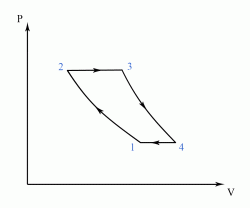Figure 3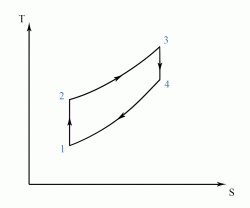Figure 4 This cycle consists of a reversible adiabatic (i.e. isentropic) compression of the WS (step ), followed by an isobaric heating (i.e. heating at constant pressure) (step ), then a reversible adiabatic (isentropic) expansion of the WS (step ), and ended with an isobaric cooling which reverts the system back to its initial state (step ).

Similar to equation (5), we can write the heat added or removed from the WS during a process at constant pressure as:

where is the number of moles of the WS, the heat capacity at constant pressure, and the change in temperature. Therefore, for the Joule cycle depicted in Figure 3 and Figure 4, the heat supplied becomes:

and the heat rejected:

Hence, the thermal efficiency of the Joule cycle becomes (see equation 4):

from which we obtain:

As for the Joule cycle the WS is also treated as pure air, we can write for the isentropic compression that:

and for the isentropic expansion that:

where is the heat capacity ratio (see equation 12).

Equations (26) and (27) can also be written as:

and,

respectively.

By raising equations (28) and (29) to the power, we respectively get that:

and,

As and (steps and of the Joule cycle are isobaric - see Figure 3), we can rewrite equations (30) and (31) as:

and,

respectively, where is called the pressure ratio.

By subtracting equation (32) from equation (33), we get that:

or furthermore, that:

By using (36) in equation (25), we obtain the thermal efficiency of the Joule cycle as:

## Diesel Cycle

The Diesel cycle is diagramed on a plot in Figure 5, and on a plot in Figure 6.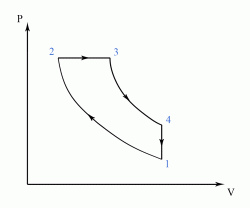Figure 5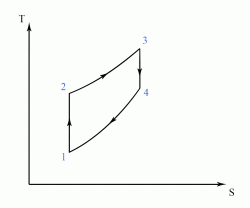Figure 6 This cycle consists of a reversible adiabatic (isentropic) compression of the WS (step ), followed by an isobaric heating (step ), then a reversible adiabatic (isentropic) expansion of the WS (step ), and ended with an isochoric cooling which returns the system back to its original state (step ).

Taking into account equations (5) and (21), we can write the heat supplied (at constant pressure, step ) as:

and the heat rejected (at constant volume, step ) as:

where is the number of moles of the WS, the heat capacity at constant pressure, and the heat capacity at constant volume.

Therefore, the thermal efficiency of the Diesel cycle becomes (see equation 4):

which can also be written as:

where is the heat capacity ratio (see equation 12).

As for the Diesel engine the WS is again treated as pure air, we can write for the isentropic compression that:

for the isobaric heating that:

and for the isentropic expansion that:

which can also be written as:

where is the compression ratio.

From equation (43) we obtain:

which can also be written as:

where is the expansion ratio during heating (also called the cut-off ratio).

The temperature from (48) can further be expressed by considering the expression of from (46), as:

which can also be written, by considering the expression of from (49), as:

We can write equation (51) in an analogous form as:

and furthermore, to:

Thus, we managed to express , , and all in function of (see equations 46, 49, and 54 respectively). By using these expression forms in equation (41), we get:

from which we obtain the thermal efficiency of the Diesel cycle as:

It can be noted that the ignition for the Diesel internal combustion engines is done by using a higher compression of the fuel, rather than by using a spark plug as in the case of gasoline powered Otto cycle engines. Therefore, the ignition for Diesel engines is sometimes called a "slow speed compression ignition", in contrast to the "spark ignition" of the Otto engines.

Example:
[imperial]
##### Example - Properties of a Diesel cycle
Problem
An engine operates under a Diesel cycle with a top pressure of (). The compression ratio is , and the air at the start of compression is at and . If are added during combustion and expansion in accordance to the law , the clearance volume is , the specific heat at constant pressure during combustion is , and also if we are to ignore the fuel mass, find:

A) the pressure, volume, and temperature at each change point in the cycle;

B) the work output of the cycle (in );

C) the thermal efficiency of the cycle.

The corresponding Diesel cycle is diagramed on a plot in Figure E1.

Workings
A) We are going to consider below each stage of the cycle separately.

Stage 1

We know from the hypothesis that the air at the start of compression is at and . Therefore, the initial pressure is:

while the initial temperature in kelvins () is:

We also know from the hypothesis that the compression ratio is . As the compression ratio is defined as:

we obtain the initial volume:

which becomes, by taking into account that and that is the clearance volume ():

Therefore , , and .

Stage 2

We have from the hypothesis that the top pressure is . Hence:

Also from the hypothesis we have that the clearance volume is . Thus:

By taking into account that:

we can write as:

By replacing the numerical values (see 1, 2, 5, 6, and 7), we obtain:

from which:

Therefore , , and .

Stage 3

As step of the Diesel cycle is isobaric (see Figure E1), we have that:

from which we obtain, by taking into account (6), that:

For step of the cycle we can also write that:

where is the heat supplied (), the specific heat at constant pressure (), and is the temperature difference expressed in , while is the quantity of gas which is present at a pressure of and at a temperature of . In order to calculate , we can write that:

from which:

Taking into account that:

and also replacing the other numerical values (see 1, 2, and 5), we get that:

from which we obtain:

By using the value of from (19) in equation (14), and also considering that , , and that , we get that:

or, by converting the temperature difference into kelvins, to:

As (see 11), we obtain as:

Still for step of the cycle we can write that:

from which becomes:

or, by replacing numerical values (see 7, 11, and 23):

Therefore , , and .

Stage 4

As step of the Diesel cycle is isochoric (see Figure E1), we have that:

from which we obtain, by taking into account (5), that:

As for step of the cycle we have that:

we can write:

from which becomes:

or, by replacing numerical values (see 13, 27, and 29):

From equation (33) we obtain:

We know that Therefore, we can divide equation (30) by and still obtain a constant:

Equation (35) can also be written as:

from which we obtain, for step of the cycle, that:

Hence, we can write that:

from which becomes:

or, by replacing numerical values (see 23, 27, and 29):

From equation (40) we obtain:

Therefore , , and .

B) The work output of the cycle is given by:

where (as ). In order to calculate , we can write that:

By applying the function to equation (44), we get:

from which we obtain:

or, by replacing numerical values (see 1, 5, 6, and 7):

By replacing all the numerical values in equation (42) (see 1, 5, 6, 7, 13, 27, 29, and 34), and also taking into account that , (see 48), and that , we get the work done in as:

from which we obtain:

C) We know that the thermal efficiency is defined as:

where is the work output, and the heat supplied.

As, in our case, (see 50) and , and also taking into account that , we get the thermal efficiency of the Diesel cycle as:

from which we obtain:

or, expressed as percentage:

Solution